# Resonance Dipole Moment and Bond Parameters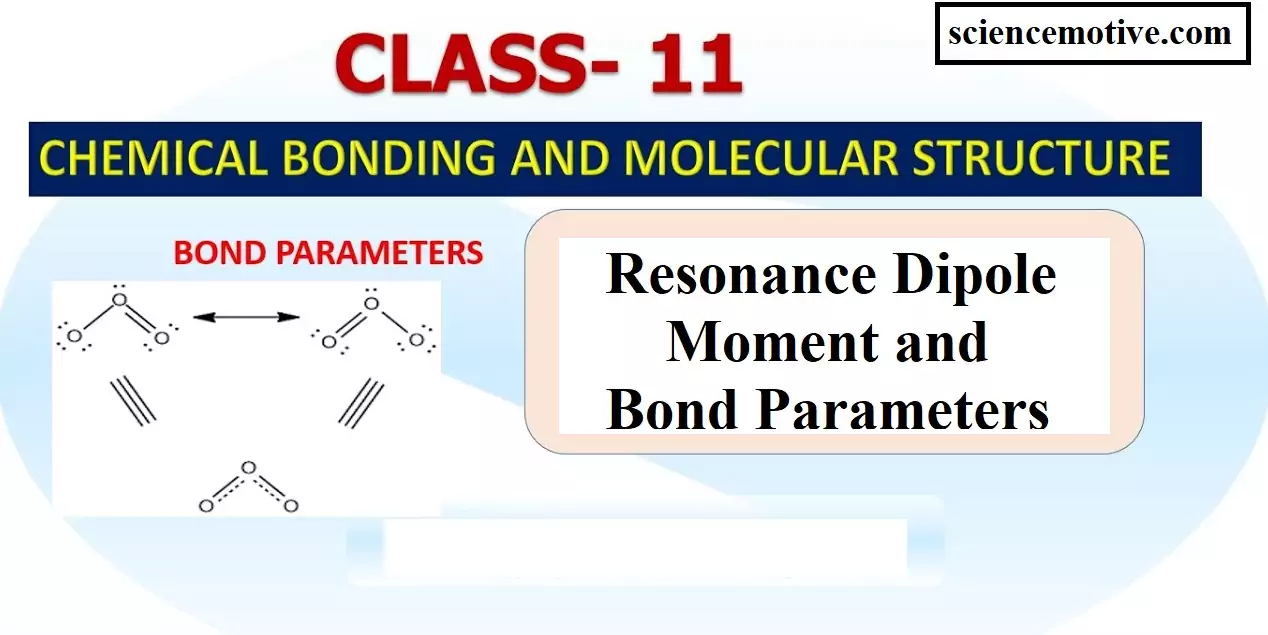Resonance Dipole Moment and Bond Parameters

Resonance Dipole Moment and Bond Parameters:

Covalent Bond Parameters:
(1) Bond Length: It is defined as the distance between the nuclei of two bonded atoms in a molecule. It is measured by spectroscopic, X-ray diffraction, and electron diffraction techniques. It is expressed in pico-meter (pm) or in the angstrom unit (A0). Bond lengths depend upon the relative sizes of the atoms. For example, the bond lengths of hydrogen halides follow the sequence H – F < H – Cl < H – Br < H – I because the size of the halogens increases down the periodic group as F < Cl < Br < I. In the case of atoms joined by multiple bonds, the greater is the bond order, the smaller will be the bond length.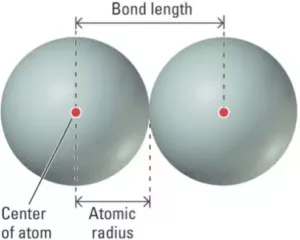(2) Bond Angle: It is defined as the angle between the orbitals containing bonding electron pairs around the central atom in a molecule. It can be experimentally determined by spectroscopic methods. It is expressed in degree.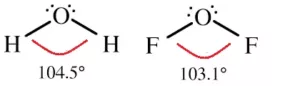(3) Bond Enthalpy (Bond Energy): Energy is released during the process of covalent bond formation between atoms which leads to the lowering of energy and energy is required to break a bond. Bond enthalpy (or bond energy) is defined as the amount of energy required to break one mole of a particular bond between 2 atoms in a gaseous state. Its unit is kJ/mol.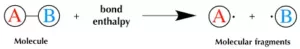(4) Bond Order: The bond order is the number of bonds present between two atoms in a molecule or ion. The bond order is said to be 1 when there is only one covalent bond formed between two atoms. For example Bond order in Cl2 and H2 is 1,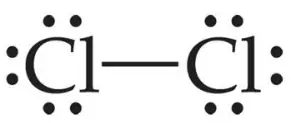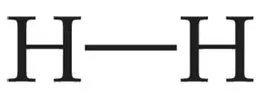Bond order for O2 is 2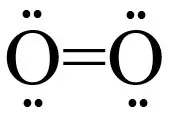Generally, with an increase in bond order, bond enthalpy increases and bond length decreases.

Dative bond or Co-ordinate bond: When in the formation of a bond between two atoms, only one atom contributes the pair of electrons and the other atom simply participates in sharing, the bond formed is called a dative bond or Co-ordinate bond. This may be explained as under:
In this case, one atom has its octet complete and has at least one lone pair (unshared) of electrons. The lone pair of electrons belonging to the atom with the complete octet is shared with the other atom containing an empty orbital and thus a link is established. The atom contributing the shared pair of electrons is called the donor while the atom which accepts these shared electrons is called the acceptor. This linkage set up between two atoms is represented by an arrow starting from the donor to the acceptor.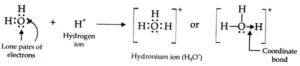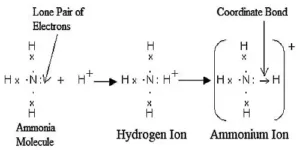Resonance and Resonating Structure: In the case of some compounds, all the observed properties cannot be explained by a single structure so we use more than one structure of the compound. These different structures are called resonance structures or canonical structures or contributing structures. The phenomenon is known as resonance. The contributing structures are written by keeping the following points in mind.
(i) In each contributing structure, the position of atoms should be the same, only the arrangement of electrons may change.
(ii) Each structure must have the same number of unpaired electrons.
(iii) The contributing structures should have energies close to each other. The contribution of a less stable contributing structure is also less.
(iv) A more stable contributing structure is one in which the negative charge resides on the most electronegative atom and a positive charge on the most electropositive atom.
(v) Various contributing structures are written with a double-headed arrow between them.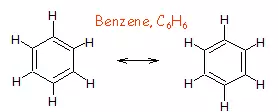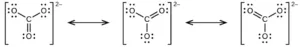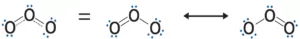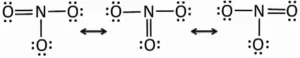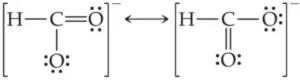Characteristics of Resonance:
(i) Resonance stabilizes the molecule. As the number of resonating structures increases, the stability also increases.
(ii) Resonance changes the bond length.
(iii) The difference in energy between the actual structure and the most stable canonical structure is called resonance energy. The greater the resonance energy, the stabler will be the molecule.

The polarity of Bonds:
(i) Non–Polar Covalent Bond: If two similar atoms come close to each other and form a bond by sharing their electrons the shared electrons are equally attracted by the atoms as their electronegativity is the same. Hence, no poles are developed. eg. Cl2, N2, O2, H2.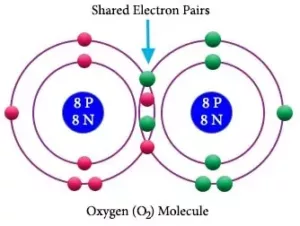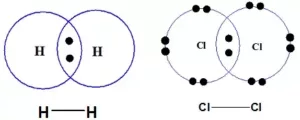(ii) Polar Covalent Bond: When two dissimilar atoms combine together to form a covalent bond, the shared pair of electrons shifts towards an atom having high electronegativity. As a result, more electronegative atom acquires a slight negative charge (δ) and the other gets a slightly positive charge (δ+). Such molecules are called polar molecules. E.g. HCl, HF, H2O, HI, etc.
The polar character of covalent bonds is measured with the help of a property called dipole moment (μ). It is defined as the product of the magnitude of charge (q) and the distance (d) between the two atoms.                             μ  =  q  x  d   Debye
The unit of dipole moment is Coulomb metre (Cm). But it is usually expressed in the unit Debye (D). 1D = 3.336 x 10-30 Cm. The dipole moment is a vector quantity. i.e. it has both magnitude and direction. It is represented by the arrow pointing towards the negative end. For eg.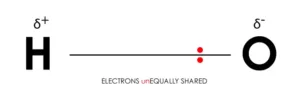In the case of polyatomic molecules, dipole moment depends on the individual bond dipoles and the spatial arrangement of bonds. Here the dipole moment of the molecule is the vector sum of the bond dipoles of various bonds.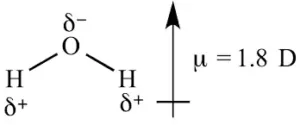For BeF2, the net dipole moment is zero, since the two equal bond dipoles are in opposite directions and cancel each other.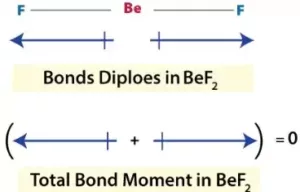In BF3, the net dipole moment is zero. Here the resultant of any 2 bond dipoles is equal and opposite to the third.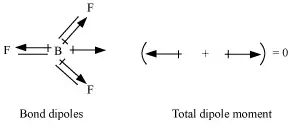Both ammonia (NH3) and nitrogen fluoride (NF3) are pyramidals in shape. Even though F is more electronegative than H, the net dipole moment of NF3 is smaller than that of NH3. This is because, in the case of NH3, the orbital dipole due to the lone pair is in the same direction as the resultant dipole moment of the three N – H bonds. But in NF3, the orbital dipole is in the opposite direction to the resultant dipole moment of the three N-F bonds. So, the dipole moments get partially canceled.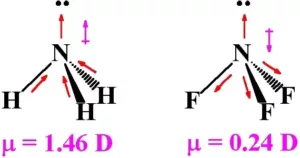Covalent Character in Ionic Compounds (Fajan’s Rule):
(i) There is no compound that is 100% ionic.
(ii) Covalent character in the ionic compound can be explained with the help of Fajan’s rule.
(iii) The smaller the size of the cation and the larger the size of the anion, the greater the covalent character of an ionic bond.
(iv) The greater the charge on the cation, the greater the covalent character of the ionic bond.
(v) (n-1)dnns0 is more polarising than the ion with a noble gas configuration (ns2 np6).

Resonance Dipole Moment and Bond Parameters